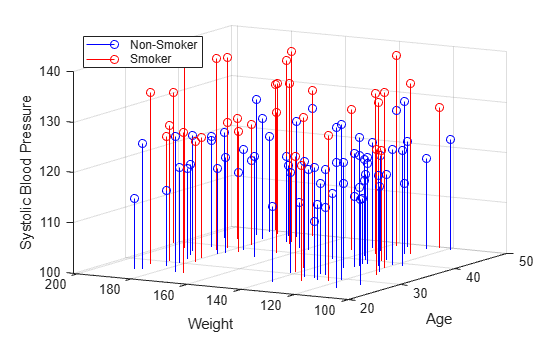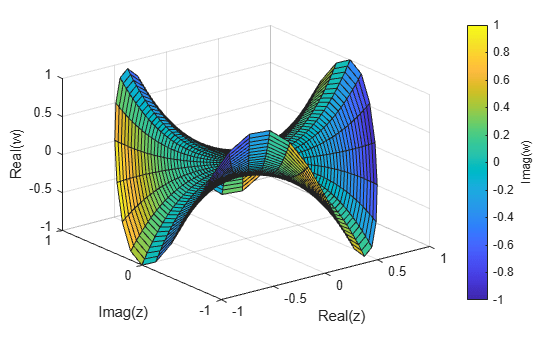# Visualizing Four-Dimensional Data

This example shows several techniques to visualize four dimensional (4-D) data in MATLAB®.

### Visualize 4-D Data with One Discrete Variable

Sometimes data has a variable which is discrete with only a few possible values. You can create multiple plots of the same type for data in each discrete group. For example, use the `stem3` function to see the relationship between three variables where the fourth variable divides the population into discrete groups.

```load patients Smoker Age Weight Systolic % load data nsIdx = Smoker == 0; smIdx = Smoker == 1; figure stem3(Age(nsIdx), Weight(nsIdx), Systolic(nsIdx), 'Color', 'b') % stem plot for non-smokers hold on stem3(Age(smIdx), Weight(smIdx), Systolic(smIdx), 'Color', 'r') % stem plot for smokers hold off view(-60,15) zlim([100 140]) xlabel('Age') % add labels and a legend ylabel('Weight') zlabel('Systolic Blood Pressure') legend('Non-Smoker', 'Smoker', 'Location', 'NorthWest')```### Visualize 4-D Data with Multiple Plots

With a large data set you might want to see if individual variables are correlated. You can use the `plotmatrix` function to create an n by n matrix of plots to see the pair-wise relationships between the variables. The `plotmatrix` function returns two outputs. The first output is a matrix of the line objects used in the scatter plots. The second is a matrix of the axes objects that are created.

The `plotmatrix` function can also be used for higher order data sets.

```load patients Height Weight Diastolic Systolic % load data labels = {'Height' 'Weight' 'Diastolic' 'Systolic'}; data = [Height Weight Systolic Diastolic]; [h,ax] = plotmatrix(data); % create a 4 x 4 matrix of plots for i = 1:4 % label the plots xlabel(ax(4,i), labels{i}) ylabel(ax(i,1), labels{i}) end```### Visualize Function of Three Variables

For many kinds of four dimensional data, you can use color to represent the fourth dimension. This works well if you have a function of three variables.

For example, represent highway deaths in the United States as a function of longitude, latitude, and if the location is rural or urban. The x, y, and z values in the plot represent these three variables. The color represents the number of highway deaths.

```cla load accidents hwydata % load data long = -hwydata(:,2); % longitude data lat = hwydata(:,3); % latitude data rural = 100 - hwydata(:,17); % percent rural data fatalities = hwydata(:,11); % fatalities data scatter3(long,lat,rural,40,fatalities,'filled') % draw the scatter plot ax = gca; ax.XDir = 'reverse'; view(-31,14) xlabel('W. Longitude') ylabel('N. Latitude') zlabel('% Rural Population') cb = colorbar; % create and label the colorbar cb.Label.String = 'Fatalities per 100M vehicle-miles';```### Visualize Data in a Volume

Your data may contain a measured value for a physical object such as temperature in a pipe. In this cases, the physical dimensions can be represented as a volume with color used to represent the magnitude of the measurement. For example, use the `slice` function to show the value of the measured variable at cross-sections within the volume.

```load fluidtemp x y z temp % load data xslice = [5 9.9]; % define the cross sections to view yslice = 3; zslice = ([-3 0]); slice(x, y, z, temp, xslice, yslice, zslice) % display the slices ylim([-3 3]) view(-34,24) cb = colorbar; % create and label the colorbar cb.Label.String = 'Temperature, C';```### Plot the Function of a Complex Variable

A complex function has an input with real and imaginary parts and an output with real and imaginary parts. You can use a three dimensional plot with color to represent the complex function. In this case the x and y axes represent the real and imaginary parts of the input. The z axis represents the real part of the output and the color represents the imaginary part of the output.

```r = (0:0.025:1)'; % create a matrix of complex inputs theta = pi*(-1:0.05:1); z = r*exp(1i*theta); w = z.^3; % calculate the complex outputs surf(real(z),imag(z),real(w),imag(w)) % visualize the complex function using surf xlabel('Real(z)') ylabel('Imag(z)') zlabel('Real(w)') cb = colorbar; cb.Label.String = 'Imag(w)';```